## Figures index

#### C. Martínez-Vera, I. Anaya-Sosa, M. G. Vizcarra-Mendoza

American Journal of Food Science and Technology. 2015, 3(5), 132-136 doi:10.12691/ajfst-3-5-3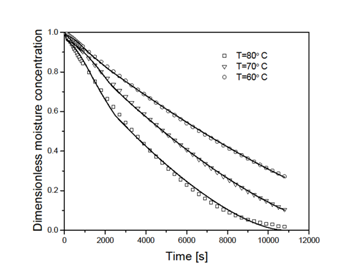• Figure 1. Experimental moisture content values (symbols) and simulated drying curves (continuous lines) obtained solving Equations (1a-1g) and Equation (2).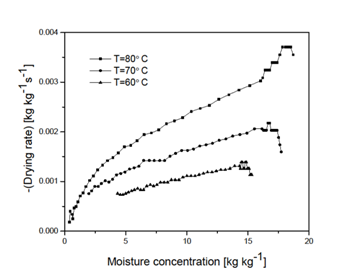• Figure 2. Drying rate versus moisture content• Figure 3. Model predictions of the radius reduction in the drying process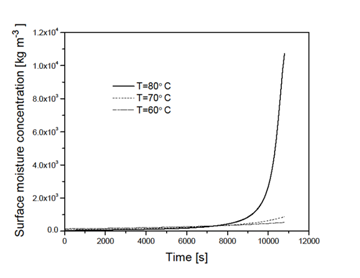• Figure 4. Evolution of the volumetric surface moisture content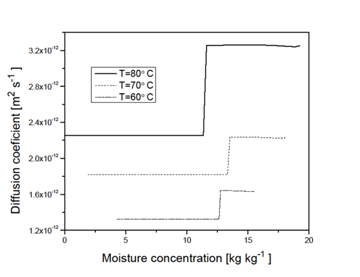• Figure 5. Spatial averaged diffusion coefficient versus moisture content.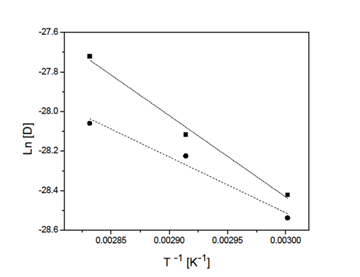• Figure 6. Fitting Arrhenius’s models to the diffusion coefficients dependency on temperature for the high (spheres) and low (triangles) moisture content ranges at each temperature level analyzed (Eah = 33.710kJ mol-1; Eal = 26.127 kJ mol-1)